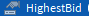# Attempt to compare two values gives error

Hey there, I am trying to compare two values to see if one is bigger then the other but it gives me the error,
` attempt to compare Instance < number`

I have a HighestBid value in my replicatedstorage which is an integerThe one comparing is a textbox input which is converted to a number,

``````local bidValue = ReplicatedStorage:WaitForChild("HighestBid")

local localBid = nil

localBid = tonumber(bidBox.Text)

if localBid > bidValue
``````

Why does it give me the error, I am pretty sure they are both integers, I tried converting bidValue to an integer but it gave me the same error.

1 Like

bidValue is just referencing the istance of the value, try doing in the compare localBid > bidValue.Value

1 Like

Oh yes, my bad lol that was very simple thank you

1 Like

You need to change `bidValue` to `bidValue.Value`:

``````local bidValue = ReplicatedStorage:WaitForChild("HighestBid")

local localBid = nil

localBid = tonumber(bidBox.Text)

if localBid > bidValue.Value
``````